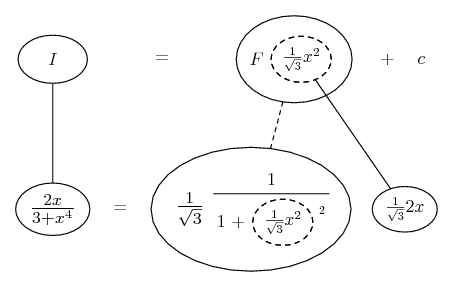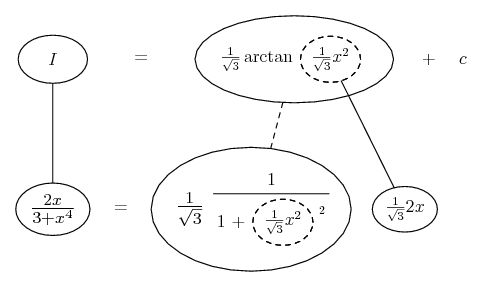# Thread: integration by substitution

1. ## integration by substitution

Integrate the following using substitution:

2x / (x^4 + 3) dx

Here's how i started:

Let u = x^4 + 3
So du = 4x^3 dx

Now I have 2x / u dx

Do I multiply 2x by 2x^2 to get 4x^3 dx and substitute in with du?
If i do this I would have to mulitply the integral by 1/2x^2, but I'm not sure if I can do this...

2. u = x^2, then a trig sub...

Edit:

(However, the trig sub is standard if you further divide x^2 by root 3, so it would have been more helpful to suggest u = x^2/root 3, as below.)

Just in case a picture helps...... where... is the chain rule. Straight continuous lines differentiate downwards (integrate up) with respect to x, and the straight dashed line similarly but with respect to the dashed balloon expression (the inner function of the composite which is subject to the chain rule).

Spoiler:_____________________________________________

Don't integrate - balloontegrate!

Balloon Calculus; standard integrals, derivatives and methods

Balloon Calculus Drawing with LaTeX and Asymptote!

3. notice that x^4 can be written as (x^2)^2. so now let u=x^2 so du=2x dx. then your integrand becomes 1 / u^2 + 3, using the formula for the integral of arctan, which is 1/a arctan(x/a),

the integral should be 1/sqrt(3) arctan(x^2 / sqrt(3)) + C.

#### Search Tags

integration, substitution